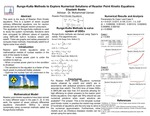## Stander Symposium Posters

#### Description

This work is the study of Reactor Point Kinetic equations. This is a system of seven coupled ordinary differential equations, one for neutron density and six for delayed neutron precursors. The application of Runge-Kutta methods is used to study the system numerically. Solutions were then compared for different values of reactivity using MATLAB built-in functions ode23 and ode45. There are graphs and tables presented to compare these methods; theoretically ode45 is of higher-order than ode23.

4-18-2018

Capstone Project

Mathematics

#### Keywords

Stander Symposium poster